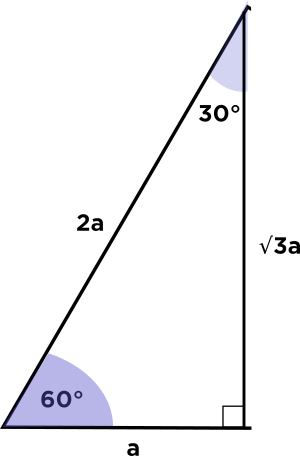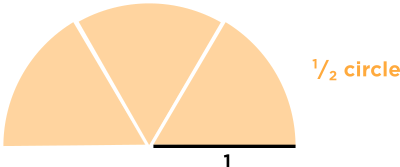# Why does Prof. Loh divide pi/2 by sqrt 3 and not something else??

• @TSS-Graviser It's because the question was asking for the ratio of the dough that goes toward the circular cookies. So we want

$$\frac{\text{area of circular wedges}}{\text{area of equilateral triangle}}$$

To find the area of the equilateral triangle, we recall that we know the ratios of the height of the equilateral triangle to its leg. In fact, that was covered in this forum post.So altitude of an equilateral triangle is equal to $$\sqrt{3} \times \frac{\text{side}}{2}$$, which means the area of the equilateral triangle is equal to

\begin{aligned} \text{ area of triangle } &= \frac{1}{2} \times \text{ base} \times \text{ height} \\ &= \frac{1}{2} \times 2 \times \sqrt{3} \\ &= \sqrt{3} \\ \end{aligned}

Inside the equilateral triangle, there are three wedges of cookie, each with an angle of $$60^{\circ}.$$Put together, the wedges make up half of a circle with radius $$1!$$Since the area of a circle with radius $$1$$ is $$\pi ,$$ the cookie area is $$\frac{\pi}{2}.$$

And since we know the area of the cookie and the area of the triangle, we can find the answer, which is the ratio of two:

$$\frac{\text{cookie area}}{\text{triangle area}} = \frac{\frac{\pi}{2}}{\sqrt{3}}$$

I hope this helps!